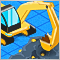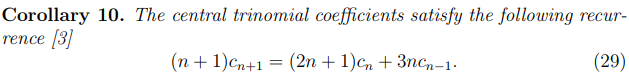# Trinomial expansion663

Let's take the following polynomial (which is closely related to the binomial expansion, and it is in fact a particular case of trinomial expansion):

(1+x+x2)n

It's expansion gives a series of coefficients, depending solely on n.

```if n = 0,            1;
if n = 1,         1  1  1;
if n = 2,      1  2  3  2  1;
if n = 3,   1  3  6  7  6  3 1;
if n = 4, 1 4 10 16 19 16 10 4 1;```
`and so on.`
So, I want to know the sum of coefficients depending on "n" and also the sum of the coefficients just above the the middle position. I was able to create a recursive formula in Microsoft Excel, but I have no clue on how to create an array out of the coefficients to give different answers, sucha as the sum below the middle element or after the middle element, such as:
```if n = 0; sum above = 0;
if n = 1; sum above = 1;
if n = 2; sum above = 3;
if n = 3; sum above = 10;
if n = 4; sum above = 31;```

and so on...

The sum of the coefficients is simply n3.

The function, I believe, should look like this:

```    int SumAbove(int n)
{
int array[][];
int j = 0;
array = 0;
for(int i=1;i<=n;i++;j++)
{
((i==0)||(i==(2*n))) ? array[i][j]=1 : array[i][j] = array[i][j-1] + array[i][j]+array[i][j+1];
}
int sum = 0;
for(int i=(n+1);i<=(2*n);i++)
{
sum =+ array[n][i]
}
return(sum);
}

```
A027907 - OEIS
• oeis.org
A027907 Irregular triangle of trinomial coefficients T(n,k) (n >= 0, 0 <= k <= 2n), read by rows (n-th row is obtained by expanding (1 + x + x^2)^n). OFFSET 0,6 COMMENTS T(n,k) = number of integer strings s(0),...,s(n) such that s(0)=0, s(n)=k, s(i) = s(i-1) + c, where c is 0, 1 or 2. Columns of...14334

Sum = 3^n.

From here663

fxsaber:

Sum = 3^n.

From here

Yes, Σ = 3n.663

fxsaber:

Sum = 3^n.

From here

But I am more interested in n, k coefficient and the sum for k<n.
```int n = 9;

int a_array[][];

a_array = 1;

for(int i=1;i<=n;i++)
{
for(int k=0;k<=(2*i);k++)
{
a_array[i][k] =
((k-2)>=0)?a_array[i-1][k-2]:0 +
((k-1)>=0)?a_array[i-1][k-1]:0 +
(k<=(2*i))?a_array[i-1][k]:0;
};
};```
Perhaps I am getting closer. Am I?663663663

Arthur Albano:
An absolutely non-parametric study of anomalies that arise from time to time in market trading. There's no such a thing as period, volume or price levels as inputs.447

I  saw  another trader trading bimodal - multimodal distributions.But how do  you trade the bimodal - multimodal distributions ?Do you  evaluate  your  trading results on multimodal trinomial distribution criteria? Do you trade  the multimodal trinomial distribution of  price?  etc..

When I google ''multimodal trinomial distribution criteria for  trading'' I can only find few  papers based on option pricing.